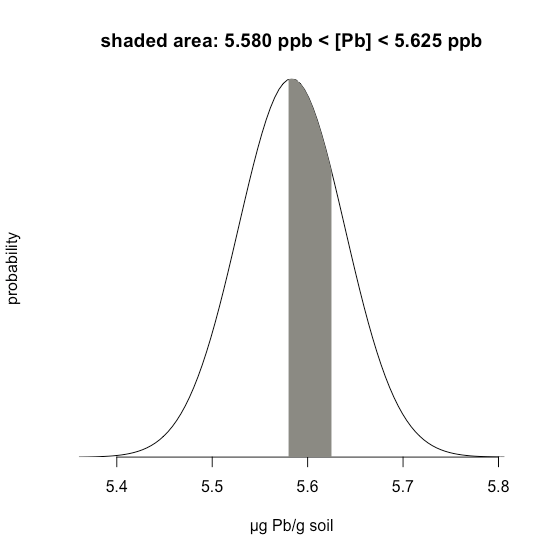# 6.1: Properties of a Normal Distribution

$$\newcommand{\vecs}{\overset { \rightharpoonup} {\mathbf{#1}} }$$ $$\newcommand{\vecd}{\overset{-\!-\!\rightharpoonup}{\vphantom{a}\smash {#1}}}$$$$\newcommand{\id}{\mathrm{id}}$$ $$\newcommand{\Span}{\mathrm{span}}$$ $$\newcommand{\kernel}{\mathrm{null}\,}$$ $$\newcommand{\range}{\mathrm{range}\,}$$ $$\newcommand{\RealPart}{\mathrm{Re}}$$ $$\newcommand{\ImaginaryPart}{\mathrm{Im}}$$ $$\newcommand{\Argument}{\mathrm{Arg}}$$ $$\newcommand{\norm}{\| #1 \|}$$ $$\newcommand{\inner}{\langle #1, #2 \rangle}$$ $$\newcommand{\Span}{\mathrm{span}}$$ $$\newcommand{\id}{\mathrm{id}}$$ $$\newcommand{\Span}{\mathrm{span}}$$ $$\newcommand{\kernel}{\mathrm{null}\,}$$ $$\newcommand{\range}{\mathrm{range}\,}$$ $$\newcommand{\RealPart}{\mathrm{Re}}$$ $$\newcommand{\ImaginaryPart}{\mathrm{Im}}$$ $$\newcommand{\Argument}{\mathrm{Arg}}$$ $$\newcommand{\norm}{\| #1 \|}$$ $$\newcommand{\inner}{\langle #1, #2 \rangle}$$ $$\newcommand{\Span}{\mathrm{span}}$$$$\newcommand{\AA}{\unicode[.8,0]{x212B}}$$

Mathematically a normal distribution is defined by the equation

$P(x) = \frac {1} {\sqrt{2 \pi \sigma^2}} e^{-(x - \mu)^2/(2 \sigma^2)} \nonumber$

where $$P(x)$$ is the probability of obtaining a result, $$x$$, from a population with a known mean, $$\mu$$, and a known standard deviation, $$\sigma$$. Figure $$\PageIndex{1}$$ shows the normal distribution curves for $$\mu = 0$$ with standard deviations of 5, 10, and 20.Figure $$\PageIndex{1}$$: Three examples of normal distribution curves. Although the height and width are affected by $$\sigma$$, the area under each curve is the same.

Because the equation for a normal distribution depends solely on the population’s mean, $$\mu$$, and its standard deviation, $$\sigma$$, the probability that a sample drawn from a population has a value between any two arbitrary limits is the same for all populations. For example, Figure $$\PageIndex{2}$$ shows that 68.26% of all samples drawn from a normally distributed population have values within the range $$\mu \pm 1\sigma$$, and only 0.14% have values greater than $$\mu + 3\sigma$$.Figure $$\PageIndex{2}$$: Normal distribution curve for $$\mu = 0$$ and $$\sigma = 1$$ showing area under the curve for various values of $$z$$ in $$\mu \pm z \sigma$$.

This feature of a normal distribution—that the area under the curve is the same for all values of $$\sigma$$—allows us to create a probability table (see Appendix 1) based on the relative deviation, $$z$$, between a limit, x, and the mean, $$\mu$$.

$z = \frac {x - \mu} {\sigma} \nonumber$

The value of $$z$$ gives the area under the curve between that limit and the distribution’s closest tail, as shown in Figure $$\PageIndex{3}$$.Figure $$\PageIndex{3}$$: Normal distribution curve for $$\mu = 0$$ and $$\sigma = 1$$ showing (on the left) the area under the curve for $$z = −1.5$$ and (on the right for $$z = +0.5$$.

## Example $$\PageIndex{1}$$

Suppose we know that $$\mu$$ is 5.5833 ppb Pb and that $$\sigma$$ is 0.0558 ppb Pb for a particular standard reference material (SRM). What is the probability that we will obtain a result that is greater than 5.650 ppb if we analyze a single, random sample drawn from the SRM?

Solution

Figure $$\PageIndex{4}$$ shows the normal distribution curve given values of 5.5833 ppb Pb for $$\mu$$ and of 0.0558 ppb Pb $$\sigma$$. The shaded area in the figures is the probability of obtaining a sample with a concentration of Pb greater than 5.650 ppm. To determine the probability, we first calculate $$z$$

$z = \frac {x - \mu} {\sigma} = \frac {5.650 - 5.5833} {0.0558} = 1.195 \nonumber$

Next, we look up the probability in Appendix 1 for this value of $$z$$, which is the average of 0.1170 (for $$z = 1.19$$) and 0.1151 (for $$z = 1.20$$), or a probability of 0.1160; thus, we expect that 11.60% of samples will provide a result greater than 5.650 ppb Pb.Figure $$\PageIndex{4}$$: Normal distribution curve for the amount of lead in a standard reference with $$\mu = 5.5833$$ ppb and $$\sigma = 0.0558$$ ppb. The shaded area shows those results for which the concentration of lead exceeds 5.650 ppb.

## Example $$\PageIndex{2}$$

Example $$\PageIndex{1}$$ considers a single limit—the probability that a result exceeds a single value. But what if we want to determine the probability that a sample has between 5.580 g Pb and 5.625 g Pb?

Solution

In this case we are interested in the shaded area shown in Figure $$\PageIndex{5}$$. First, we calculate $$z$$ for the upper limit

$z = \frac {5.625 - 5.5833} {0.0558} = 0.747 \nonumber$

and then we calculate $$z$$ for the lower limit

$z = \frac {5.580 - 5.5833} {0.0558} = -0.059 \nonumber$

Then, we look up the probability in Appendix 1 that a result will exceed our upper limit of 5.625, which is 0.2275, or 22.75%, and the probability that a result will be less than our lower limit of 5.580, which is 0.4765, or 47.65%. The total unshaded area is 71.4% of the total area, so the shaded area corresponds to a probability of

$100.00 - 22.75 - 47.65 = 100.00 - 71.40 = 29.6 \% \nonumber$Figure $$\PageIndex{5}$$: Normal distribution curve for the amount of lead in a standard reference with $$\mu = 5.5833$$ ppb and $$\sigma = 0.0558$$ ppb. The shaded area shows those results for which the concentration of lead is more than 5.580 ppb and less than 5.625 ppb.

This page titled 6.1: Properties of a Normal Distribution is shared under a CC BY-NC-SA 4.0 license and was authored, remixed, and/or curated by David Harvey.